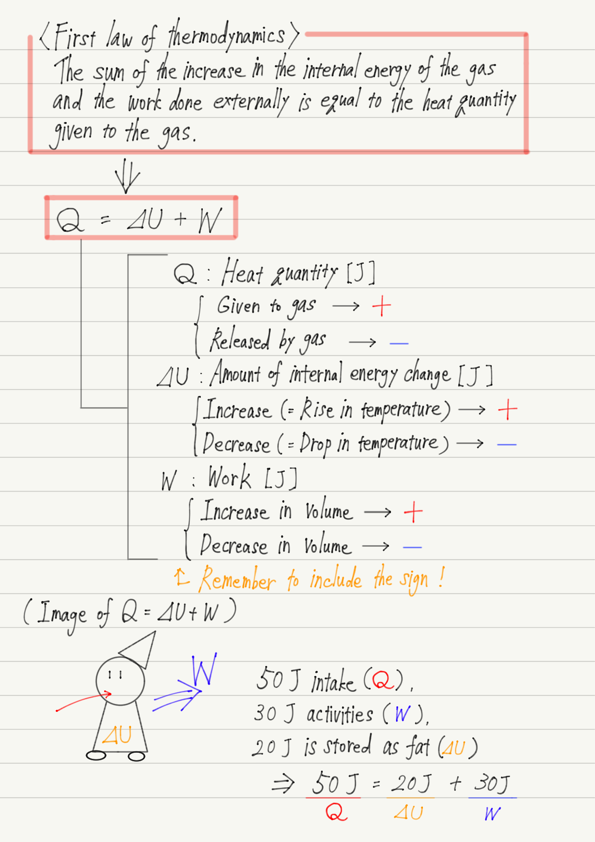# First Law of Thermodynamics(2)

I would like to explain the contents of the first law of thermodynamics based on the previous lecture.

https://www.yukimura-physics.com/entry/therm-f07

## Expression of the first law of thermodynamics

The first law of thermodynamics, using Q, U, and W introduced in the previous section, is expressed as follows.

Q = ⊿U + W

High school physics is full of proportionality formulas, so formulas written in addition like this are rare.
Note that , which represents the amount of change, is attached to U.
（In other words,⊿U=Change in internal energy）

The equation is very simple, but what does it mean?

## Meaning of the first law of thermodynamics

This equation shows “how heat is used when heat is given to an object” (I wrote “object,” but I will continue to speak of a gas).

When asked the question, “What happens when you apply heat to a gas?”
I think most people would answer, “The temperature rises.”
⊿U corresponds to this “rise in temperature” .
(According to the previous lecture, as temperature rises, internal energy also increases.).

There is another answer to the above question. It is that “gas expands”.
When you heat up bagged food in the microwave, the bag swells up, right?

In physical terms, “a gas expands” is “a gas doing work externally.
W is to respond to this work.

In light of the above, the first law of thermodynamics can be interpreted as follows.

When heat is applied to a gas, the heat is used to rise its temperature (increase in internal energy) and to expand its volume (work done externally).
The sum of the amount used for the temperature rise and the amount used for the expansion is equal to the heat quantity given at the beginning.

The important part is the second half. The energy used equals the thermal energy given.
This means that we can sum it up in one phrase: “Energy is conserved.”

## Image of the first law of thermodynamics

The first law is easy to remember when compared to humans.
We consume energy by eating food, which corresponds to Q in the first law.

Then, we use the energy we eat and ingest to perform activities (i.e., work), which corresponds to W.

By the way, if you work as much as you eat, all the energy you ingest will be expended, but what happens if you sleep without working?

Yes, you will get fat!
The portion of energy ingested that is not used for work becomes fat.
Since fat is the energy stored in the body, let us compare this to an increase in internal energy ⊿U.

In summary, the first law of thermodynamics can be interpreted as follows when compared to humans.

Q(Energy from eating)＝⊿U(Energy stored as fat)＋W（Energy of activity）

In this light, the first law of thermodynamics seems to assert the obvious.

## Notes on calculation

The most important thing to keep in mind when using the first law of thermodynamics is the sign.
For example, if we say “50 J of heat was added to the gas,” we would substitute Q = 50, but if we say “50 J of heat was released,” we must substitute -50 for Q.

Note that you have to add a minus sign if necessary in this way!

## Summary of this lecture## Next Time

In the next lecture, we will talk about a device that converts heat into work.

https://www.yukimura-physics.com/entry/therm-f09

error: Content is protected !!